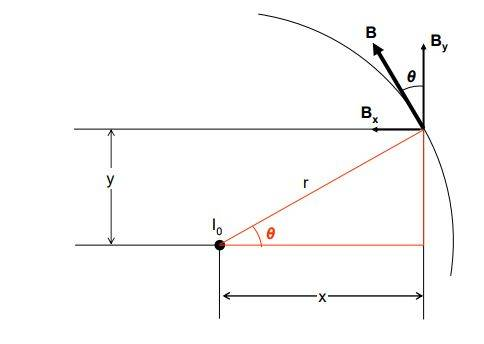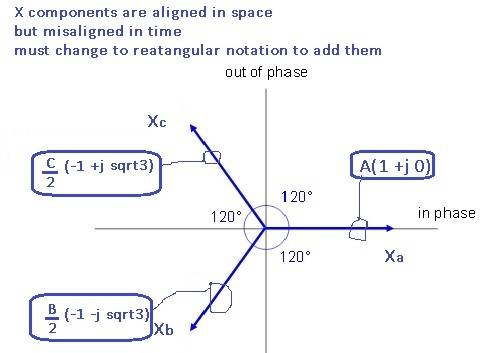# Ampere's law to calculate the B field from a balanced three-phase system

anorlunda
Staff Emeritus
@anorlunda Very good. If I interpret what you are saying correctly: Are you saying that the magnetic fields from one wire cause magnetic fields at the other wires (cables), so that it is impossible to run as much current down each phase of a 3-phase system as you can down a single wire of single phase system without getting power losses caused by these magnetic fields?

Good try but not quite. A three phase circuit carries 3x the power of a two wire single phase circuit. I'm asking you to compare one three phase circuit (3 wires) with double circuit (6 wires) on the same tower.

Homework Helper
Gold Member
2020 Award
Good try but not quite. A three phase circuit carries 3x the power of a two wire single phase circuit. I'm asking you to compare one three phase circuit (3 wires) with double circuit (6 wires) on the same tower.
@anorlunda I just spotted my mistake also. See my edited post #23 above.

•anorlunda
anorlunda
Staff Emeritus
@anorlunda I just spotted my mistake also. See my edited post #23 above.

Yes. Now you got it. The fields of the two three phase circuits must interact with each other. If the external B field was zero, as in the OP, they wouldn't.

Edit: IMO that's another example of applying common sense alongside so-called absolute truths like Amperes law.

•Asymptotic, jim hardy and Charles Link
jim hardy
Gold Member
Dearly Missed
Yes. Now you got it. The fields of the two three phase circuits must interact with each other.
I'd have never thought about that. Voltage drop along a line is small so i see how coupling could have a surprising effect.

Ahh, big machines are just full of surprises.

old jim

•Homework Helper
Gold Member
2020 Award
I'd have never thought about that. Voltage drop along a line is small so i see how coupling could have a surprising effect.

Ahh, big machines are just full of surprises.

old jim
In this case, (@anorlunda and @jim hardy please correct me if I'm wrong), I believe it is actually the change in magnetic field over time (by being sinusoidal at 60 Hz) that generates a Faraday E.M.F., ## \mathcal{E}=-d \Phi_m/dt ##, that is responsible for much of the power loss. The current that travels through this E.M.F. experiences a power loss which is essentially ## P=\mathcal{E} I ##. ## \\ ## (And perhaps it is slightly more complicated than this, because this would tend to indicate that sometimes a stray ## \mathcal{E} ## could be beneficial. A complete analysis might involve transmission line (wave) equations, and that would be kind of digressing off topic.)

Last edited:
jim hardy
Gold Member
Dearly Missed
Power transmission is really out of my field

but applying basics it would seem to me related to mutual inductance, ie the proximity of the lines lets them couple flux raising apparent impedance..

So it's not a power loss, that would make heat
but an increase in reactance that would require more volts to push along the same power.

I anticipate a brief introductory lesson from somebody who's experienced in that field....

old jim

Last edited:
Homework Helper
Gold Member
2020 Award
Power transmission is really out of my field

but applying basics it would seem to me related to mutual inductance, ie the proximity of the lines lets them couple flux raising apparent impedance..

So it's not a power loss, that would make heat
but an increase in reactance would require more volts to push along same power.

I anticipate a brief introductory lesson from somebody who's experienced in that field....

old jim
Yes @jim hardy I think I could also use a little more introductory material in regard to how the power transmission lines work that keep the power going in all of our cities. It is so easy to just flip a switch and the lights go on, but the reason it is so easy is that plenty of people have worked very hard to figure out a lot of details that make it work so well. :) :)

Bavuka
Hi all, good thread for a newbie like me. I studied the document @jim hardy posted in post #6 and got one question to ask regarding the document.

Everything is clear until page 4, up til they write "We now need to resolve each current into an in-phase and an out-of-phase component. We do this using a phasor diagram:" Why is this step necessary?

We can calculate the magnitude of $\vec{B}(t)$ at point P using:

$B_n(t) = \frac{\mu _0 i_n(t)}{2\pi r_p}$​

We now have the magnitude of $\vec{B}(t)$ (see figure below, which is from the pdf document linked in post #6)
To get $B_x(t)$ and $B_y(t)$, we do the following:

$B_{y}(t) = \vec{B}(t) cos(\theta)$ and $-B_x(t) = \vec{B}(t) sin(\theta)$ (minus sign based on the figure)​

Now we have the x and y component of $B_y(t)$ from lets say phase A, we can do the same for phase B and C, just remember to use proper distance $r$, proper angle $\theta$ and proper value of $B_{a,b,c}$.

To get the magnitude we can do:
$\vec{B}_{resultant}(t) = \vec{B}_a(t) + \vec{B}_b(t) + \vec{B}_c(t)$
or if we just care about the magnitude and not direction
$B_{resultant}(t) = \sqrt{(B_{a,x}(t)+B_{b,x}(t)+B_{c,x}(t))^2 + (B_{a,y}(t)+B_{b,y}(t)+B_{c,y}(t))^2}$

Doing it like this I do not have to worry about resolving each current into an in-phase and an out-of-phase component like shown in the pdf document.

Appreciated if someone could look over my calculations and tell me if this resolving of currents is necessary or not.

Thanks#### Attachments

Homework Helper
Gold Member
2020 Award
@Bavuka The phasor diagram method can perhaps be better explained with trigonometric calculations: ## \\ ## If we begin with ## A cos(\omega t)+Bcos(\omega t+\phi)=Acos(\omega t)+B(cos(\omega t)cos(\phi)-sin(\omega t)sin(\phi)) ## which can be written in the form ## S=Ccos(\omega t)+Dsin(\omega t) ## by grouping terms. There is an algebraic trick that is used next: ## \\ ## ## S=\sqrt{C^2+D^2} [cos(\omega t) \frac{C}{\sqrt{C^2+D^2}}+sin(\omega t) \frac{D}{\sqrt{C^2+D^2}}]##. ## \\ ## This can be written as ## S=Ecos(\omega t-\theta) ## where ## E=\sqrt{C^2+D^2} ## with ## cos(\theta)=\frac{C}{\sqrt{C^2+D^2}} ## and ## sin (\theta)=\frac{D}{\sqrt{C^2+D^2}} ## so that ## tan(\theta)=D/C ##. (Recall the trigonometric identity for the expansion of ## cos(\omega t-\theta) ##).## \\ ## The process here to do this calculation was to divide the terms up into ## cos(\omega t) ## and ## sin(\omega t) ##. We did it for just two ## cos(\omega t +\phi) ## terms, (the first one has ## \phi=0 ##), but you could do it for any number of them. In the problem at hand (the three phase electrical system with ## \omega =2 \pi f ## and ## f=60 \, Hz ##), we have 3 signals: one with ## \phi=0 ##, one with ## \phi=\pi/3 ##, and one with ## \phi=2 \pi/3 ##.

Last edited:
•Bavuka and jim hardy
anorlunda
Staff Emeritus
Ok, now for the tiresome lesson.Power can be lost in transmission only via resistance. ##I^2R##

There are several ways to calculate the max power transfer of a transmission line.

1. One is the thermal limit proportional to those ##I^2R## losses and ambient temperature. High loads on hot days make the lines sag. If they sag too much they short out on tree branches. That's what started the 2003 blackout in Ohio.
2. Stability limits are related to the series reactance in complicated ways. Reactance can be changed by mutual coupling to nearby lines. (Which is the point relevant to this thread)

Which limit is most limiting depends mostly on the length of the line.

Fun thread.•jim hardy
Gold Member
Dearly Missed
Everything is clear until page 4, up til they write "We now need to resolve each current into an in-phase and an out-of-phase component. We do this using a phasor diagram:" Why is this step necessary?

[ I haven't forgot you, just this one was going to take some study and i had to use daylight to finish putting side back on the barn. ]

I think Charles did a better job with the algebra and Latex than i could.

It's difficult to add in polar notation . I don't know how to do it.

One of the three flux's X components is of the form Asin(ωt) , one is Bsin(ωt-120°) and one is Csin(ωt+120°).
A, B and C differ because of the different radii from observer to each phase of the transmission line..
likewise their Y components.

To get the magnitude we can do:
⃗Bresultant(t)=⃗Ba(t)+⃗Bb(t)+⃗Bc(t)
once for X components and once for Y components ? Sure,
but because they're 120 degrees apart
I'd have to convert them EDIT to polar from polar to rectangular notation to add them.(please pardon misspelling of rectangular , )

same exercise for Y components.

Maybe you know some math shortcut that i don't.

old jim

Last edited:
•Bavuka
rude man
Homework Helper
Gold Member
Thanks for the replies. I looked at a table which showed typical values of B at a distance x from a 420 kV transmission line, and they were not zero. So if I would want to calculate the value of B below the transmission line at ground level, I would have to use Biot-Savart equation on each conductor and sum them up?
Yes.
Ampere's law gives the total integral of the B field (actually B⋅ds) surrounding one or more current-carrying wires. But if the wires are separated you lose symmetry and the value of B will vary as you go around any amperian loop.

•jim hardy and (deleted member)